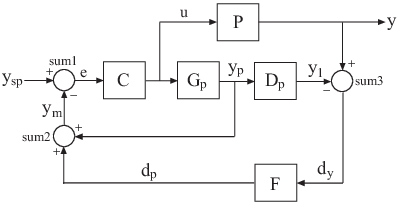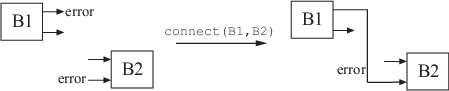## Multi-Loop Control System

This example shows how to build an arbitrary block diagram by connecting models using `connect`. The system is a Smith Predictor, the single-input, single-output (SISO) multi-loop control system shown in the following block diagram.The `connect` command lets you construct the overall transfer function from ysp to y. To use `connect`, specify the input and output channel names of the components of the block diagram. `connect` automatically joins ports that have the same name, as shown in the following figure.To build the closed loop model of the Smith Predictor system from ysp to y:

1. Create the components of the block diagram: the process model `P`, the predictor model `Gp`, the delay model `Dp`, the filter `F`, and the PI controller `C`. Specify names for the input and output channels of each model so that `connect` can automatically join them to build the block diagram.

```s = tf('s'); P = exp(-93.9*s) * 5.6/(40.2*s+1); P.InputName = 'u'; P.OutputName = 'y'; Gp = 5.6/(40.2*s+1); Gp.InputName = 'u'; Gp.OutputName = 'yp'; Dp = exp(-93.9*s); Dp.InputName = 'yp'; Dp.OutputName = 'y1'; F = 1/(20*s+1); F.InputName = 'dy'; F.OutputName = 'dp'; C = pidstd(0.574,40.1); C.Inputname = 'e'; C.OutputName = 'u';```
2. Create the summing junctions needed to complete the block diagram.

```sum1 = sumblk('e = ysp - ym'); sum2 = sumblk('ym = yp + dp'); sum3 = sumblk('dy = y - y1');```

The argument to `sumblk` is a formula that relates the input and output signals of the summing junction. `sumblk` creates a summing junction with the input and output signal names specified in the formula. For example, in `sum1`, the formula ```'e = ysp - ym'``` specifies an output signal named `e`, which is the difference between input signals named `ysp` and `ym`.

3. Assemble the complete model from ysp to y.

`T = connect(P,Gp,Dp,C,F,sum1,sum2,sum3,'ysp','y');`

You can list the models and summing junctions in any order because `connect` automatically interconnects them using their input and output channel names.

The last two arguments specify the input and output signals of the multi-loop control structure. Thus, `T` is a `ss` model with input `ysp` and output `y`.